# Fundamentals of ThermalFluid Sciences 4 th Edition in

• Slides: 31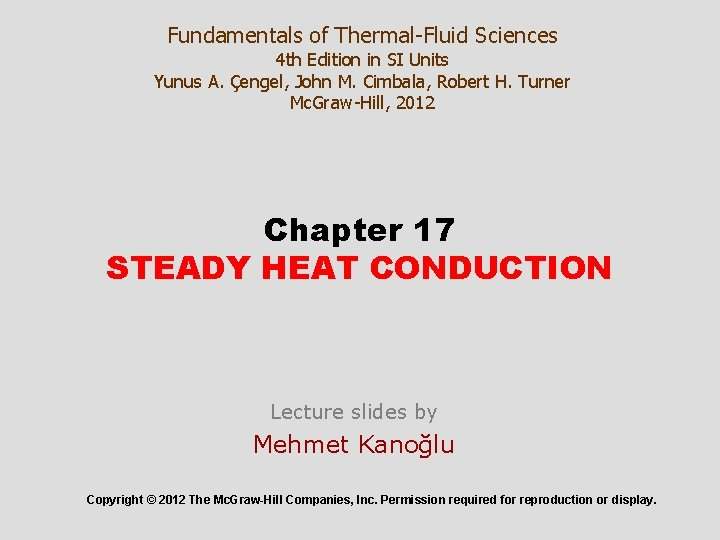Fundamentals of Thermal-Fluid Sciences 4 th Edition in SI Units Yunus A. Çengel, John M. Cimbala, Robert H. Turner Mc. Graw-Hill, 2012 Chapter 17 STEADY HEAT CONDUCTION Lecture slides by Mehmet Kanoğlu Copyright © 2012 The Mc. Graw-Hill Companies, Inc. Permission required for reproduction or display.Objectives • Understand the concept of thermal resistance and its limitations, and develop thermal resistance networks for practical heat conduction problems • Solve steady conduction problems that involve multilayer rectangular, cylindrical, or spherical geometries • Develop an intuitive understanding of thermal contact resistance, and circumstances under which it may be significant • Identify applications in which insulation may actually increase heat transfer • Analyze finned surfaces, and assess how efficiently and effectively fins enhance heat transfer 2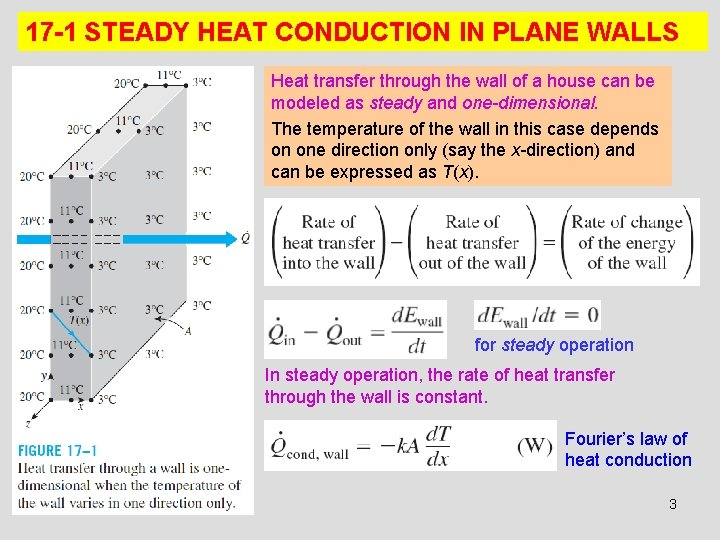17 -1 STEADY HEAT CONDUCTION IN PLANE WALLS Heat transfer through the wall of a house can be modeled as steady and one-dimensional. The temperature of the wall in this case depends on one direction only (say the x-direction) and can be expressed as T(x). for steady operation In steady operation, the rate of heat transfer through the wall is constant. Fourier’s law of heat conduction 3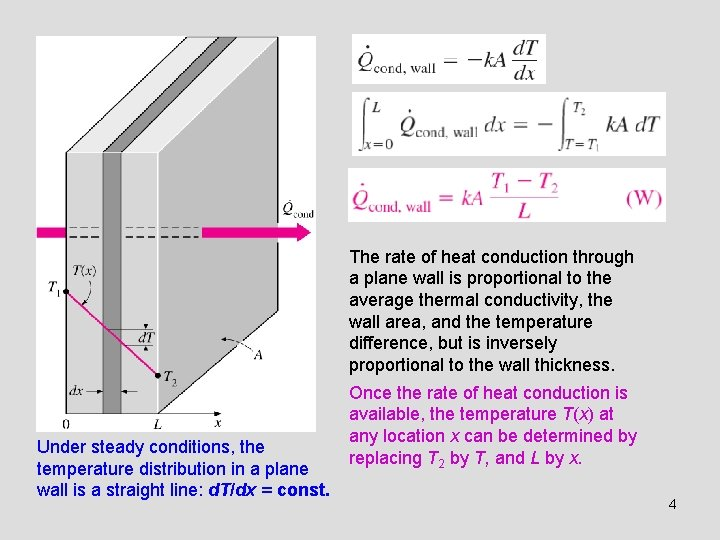The rate of heat conduction through a plane wall is proportional to the average thermal conductivity, the wall area, and the temperature difference, but is inversely proportional to the wall thickness. Under steady conditions, the temperature distribution in a plane wall is a straight line: d. T/dx = const. Once the rate of heat conduction is available, the temperature T(x) at any location x can be determined by replacing T 2 by T, and L by x. 4Thermal Resistance Concept Conduction resistance of the wall: Thermal resistance of the wall against heat conduction. Thermal resistance of a medium depends on the geometry and thermal properties of the medium. Electrical resistance Analogy between thermal and electrical resistance concepts. rate of heat transfer electric current thermal resistance electrical resistance temperature difference voltage difference 5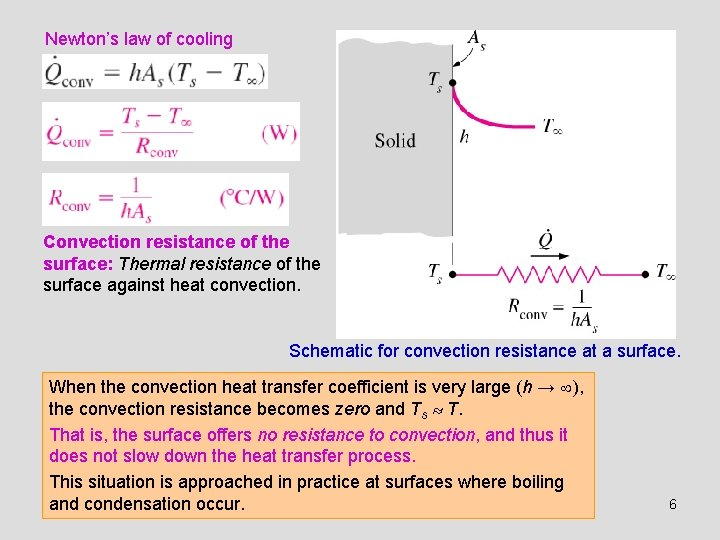Newton’s law of cooling Convection resistance of the surface: Thermal resistance of the surface against heat convection. Schematic for convection resistance at a surface. When the convection heat transfer coefficient is very large (h → ), the convection resistance becomes zero and Ts T. That is, the surface offers no resistance to convection, and thus it does not slow down the heat transfer process. This situation is approached in practice at surfaces where boiling and condensation occur. 6Radiation resistance of the surface: Thermal resistance of the surface against radiation. Radiation heat transfer coefficient Combined heat transfer coefficient Schematic for convection and radiation resistances at a surface. 7Thermal Resistance Network The thermal resistance network for heat transfer through a plane wall subjected to convection on both sides, and the electrical analogy. 8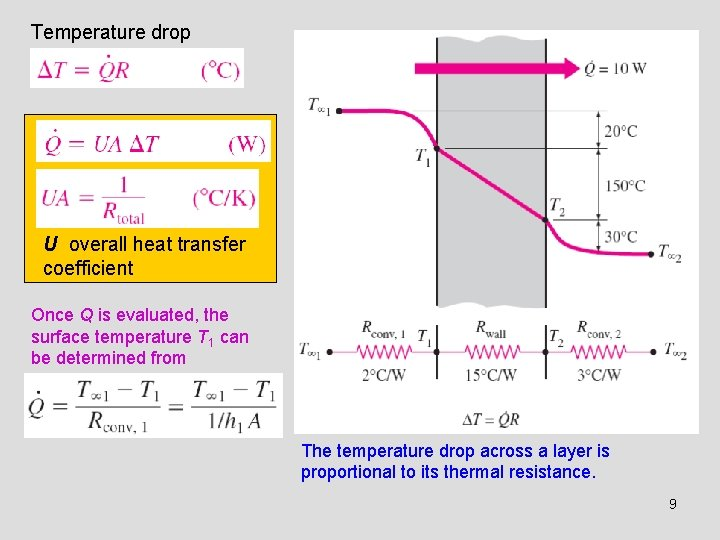Temperature drop U overall heat transfer coefficient Once Q is evaluated, the surface temperature T 1 can be determined from The temperature drop across a layer is proportional to its thermal resistance. 9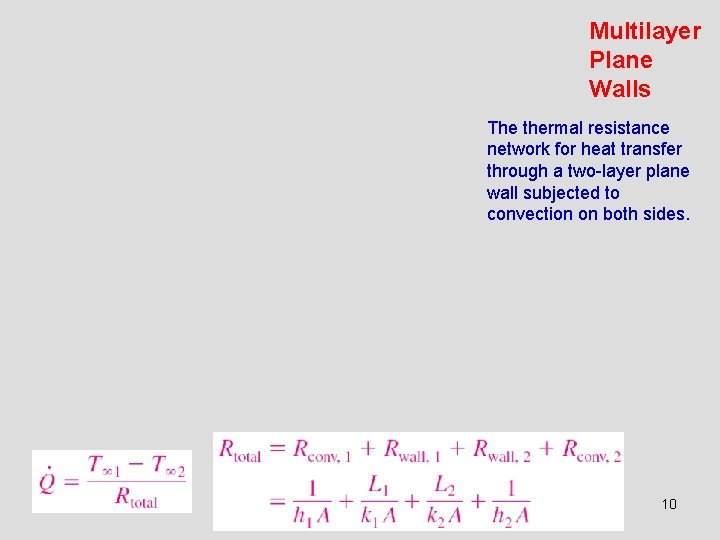Multilayer Plane Walls The thermal resistance network for heat transfer through a two-layer plane wall subjected to convection on both sides. 1011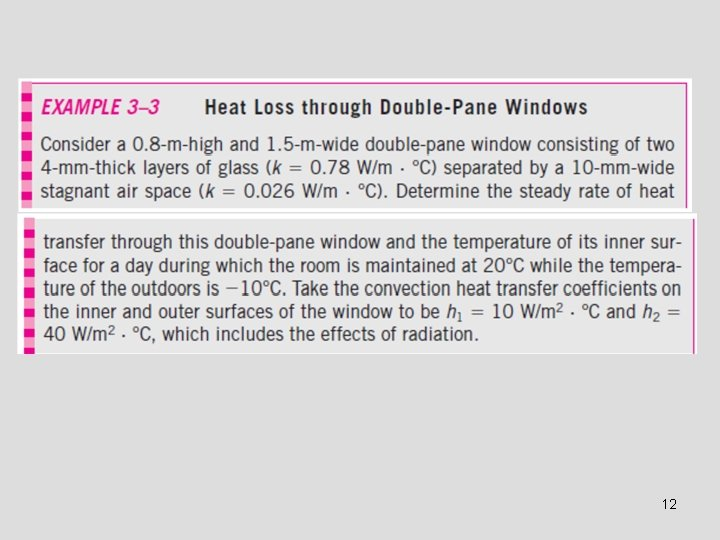1217 -2 THERMAL CONTACT RESISTANCE Temperature distribution and heat flow lines along two solid plates pressed against each other for the case of perfect and imperfect contact. 13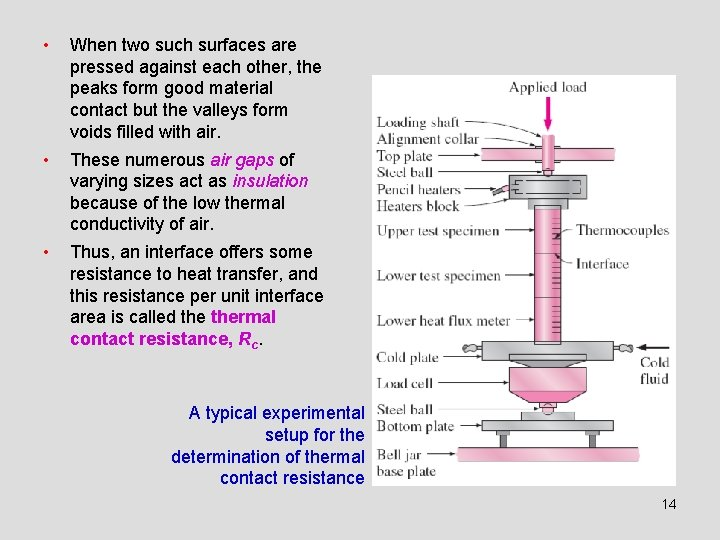• When two such surfaces are pressed against each other, the peaks form good material contact but the valleys form voids filled with air. • These numerous air gaps of varying sizes act as insulation because of the low thermal conductivity of air. • Thus, an interface offers some resistance to heat transfer, and this resistance per unit interface area is called thermal contact resistance, Rc. A typical experimental setup for the determination of thermal contact resistance 14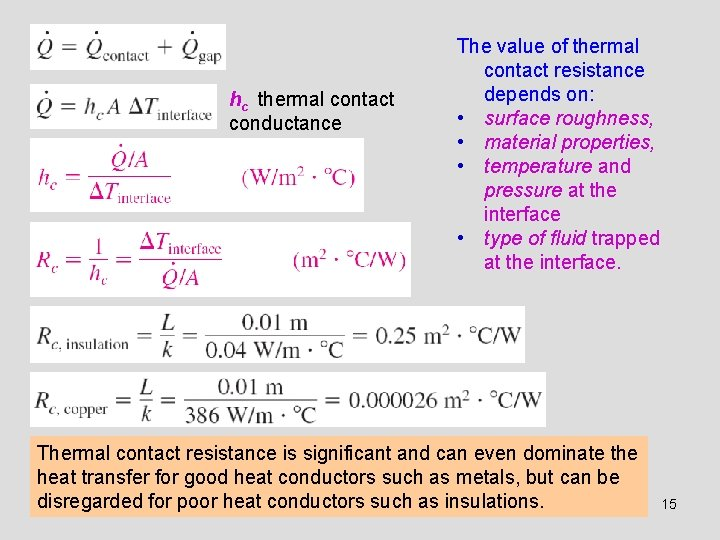hc thermal contact conductance The value of thermal contact resistance depends on: • surface roughness, • material properties, • temperature and pressure at the interface • type of fluid trapped at the interface. Thermal contact resistance is significant and can even dominate the heat transfer for good heat conductors such as metals, but can be disregarded for poor heat conductors such as insulations. 15The thermal contact resistance can be minimized by applying • a thermal grease such as silicon oil • a better conducting gas such as helium or hydrogen • a soft metallic foil such as tin, silver, copper, nickel, or aluminum Effect of metallic coatings on thermal contact conductance 16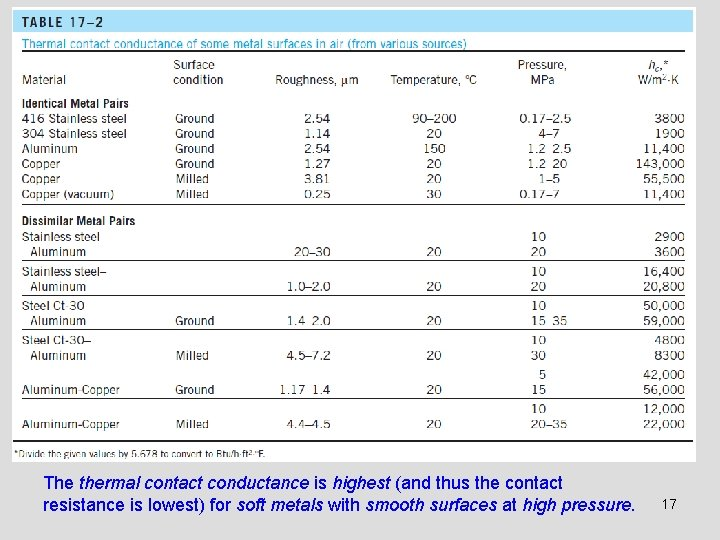The thermal contact conductance is highest (and thus the contact resistance is lowest) for soft metals with smooth surfaces at high pressure. 17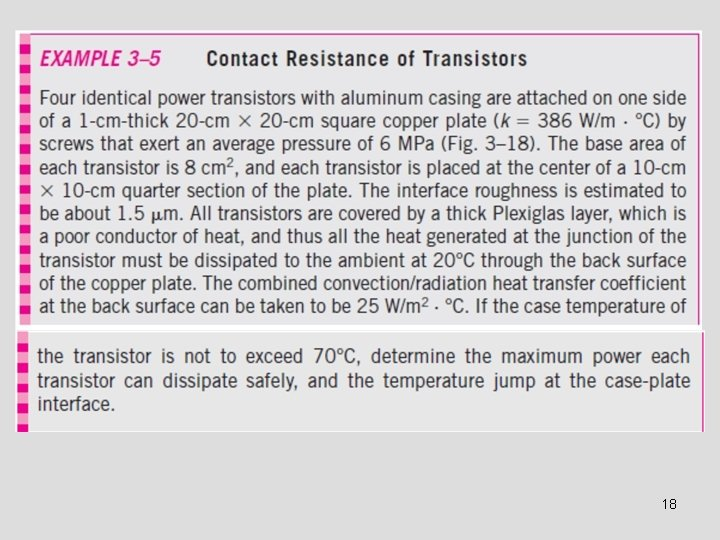18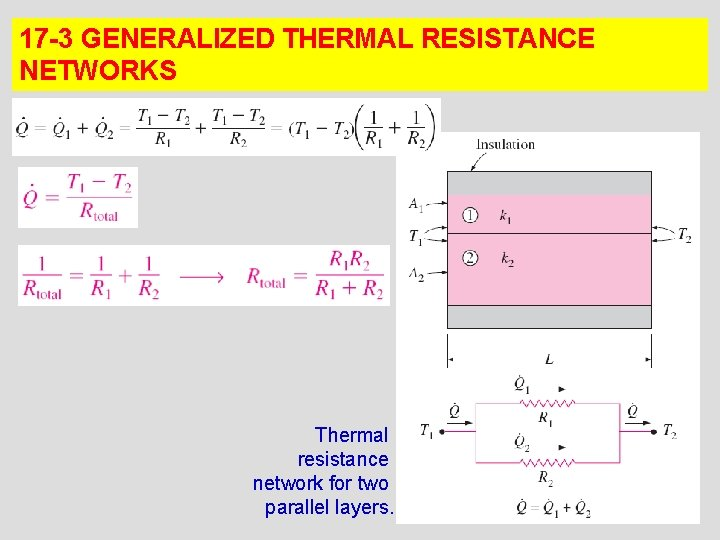17 -3 GENERALIZED THERMAL RESISTANCE NETWORKS Thermal resistance network for two parallel layers. 19Two assumptions in solving complex multidimensional heat transfer problems by treating them as one-dimensional using thermal resistance network are (1) any plane wall normal to the x-axis is isothermal (i. e. , to assume the temperature to vary in the x-direction only) (2) any plane parallel to the x-axis is adiabatic (i. e. , to assume heat transfer Thermal resistance network for to occur in the x-direction only) combined series-parallel Do they give the same result? arrangement. 20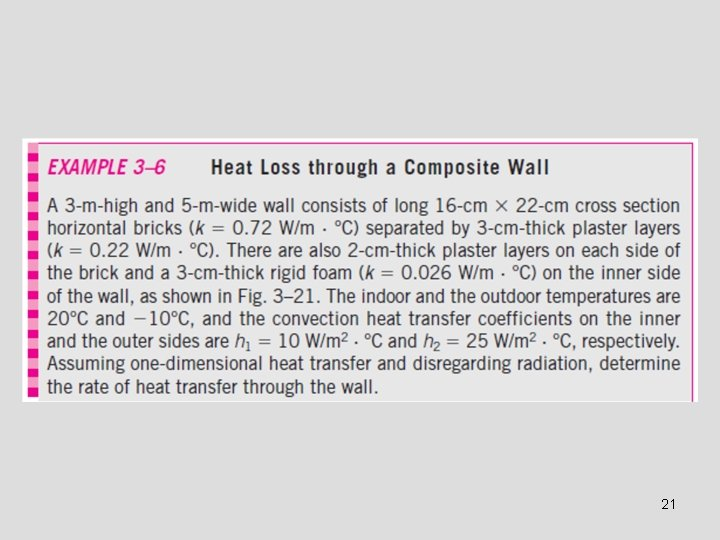21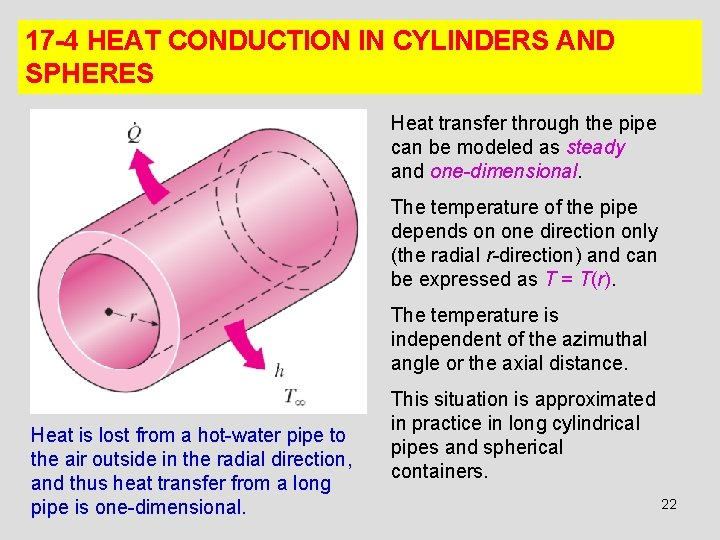17 -4 HEAT CONDUCTION IN CYLINDERS AND SPHERES Heat transfer through the pipe can be modeled as steady and one-dimensional. The temperature of the pipe depends on one direction only (the radial r-direction) and can be expressed as T = T(r). The temperature is independent of the azimuthal angle or the axial distance. Heat is lost from a hot-water pipe to the air outside in the radial direction, and thus heat transfer from a long pipe is one-dimensional. This situation is approximated in practice in long cylindrical pipes and spherical containers. 22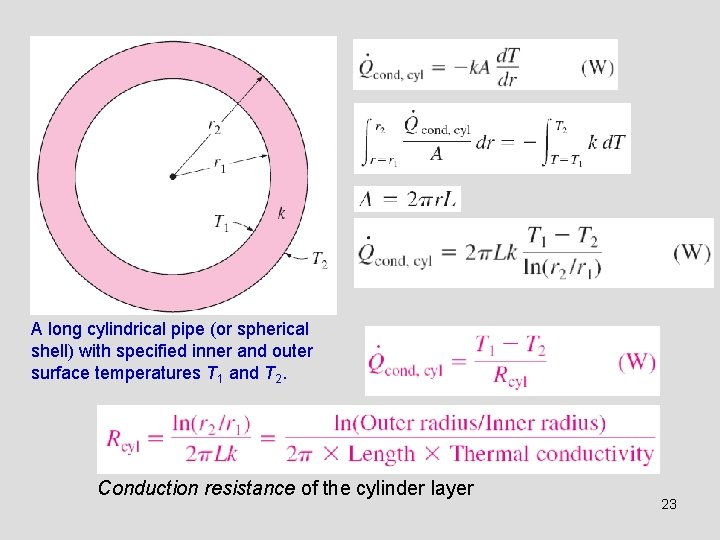A long cylindrical pipe (or spherical shell) with specified inner and outer surface temperatures T 1 and T 2. Conduction resistance of the cylinder layer 23A spherical shell with specified inner and outer surface temperatures T 1 and T 2. Conduction resistance of the spherical layer 24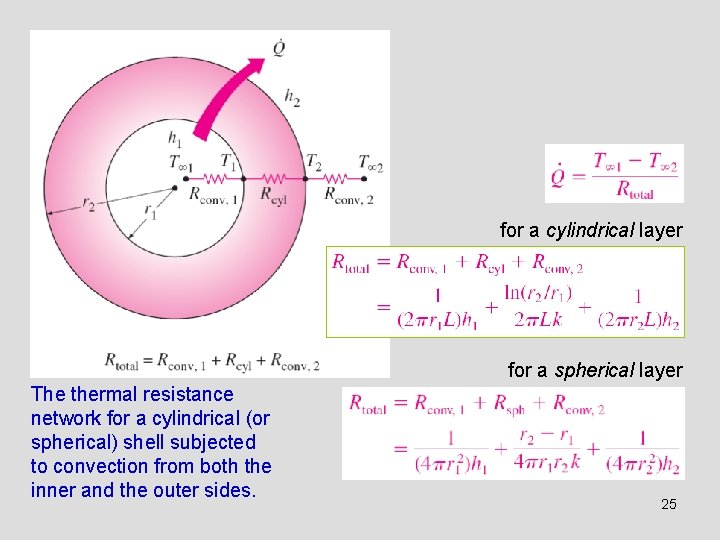for a cylindrical layer for a spherical layer The thermal resistance network for a cylindrical (or spherical) shell subjected to convection from both the inner and the outer sides. 25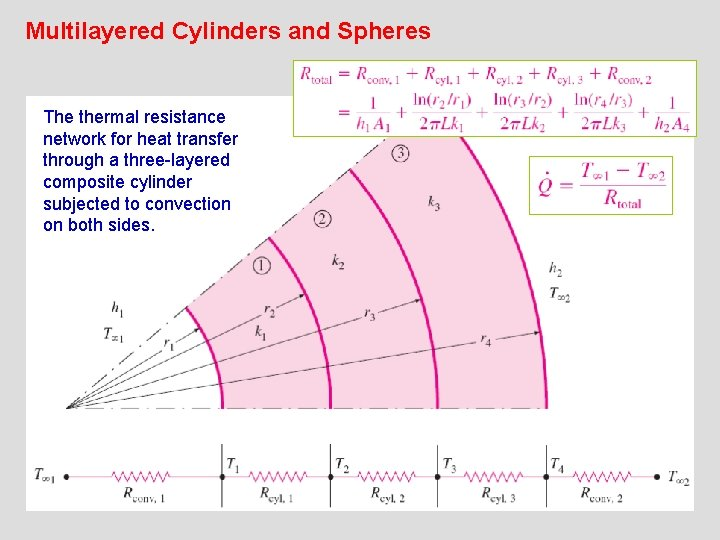Multilayered Cylinders and Spheres The thermal resistance network for heat transfer through a three-layered composite cylinder subjected to convection on both sides. 26Once heat transfer rate Q has been calculated, the interface temperature T 2 can be determined from any of the following two relations: 272817 -5 CRITICAL RADIUS OF INSULATION Adding more insulation to a wall or to the attic always decreases heat transfer since the heat transfer area is constant, and adding insulation always increases thermal resistance of the wall without increasing the convection resistance. In a a cylindrical pipe or a spherical shell, the additional insulation increases the conduction resistance of the insulation layer but decreases the convection resistance of the surface because of the increase in the outer surface area for convection. An insulated cylindrical pipe exposed to convection from the outer surface and thermal resistance network associated with it. The heat transfer from the pipe may increase or decrease, depending on which effect dominates. 29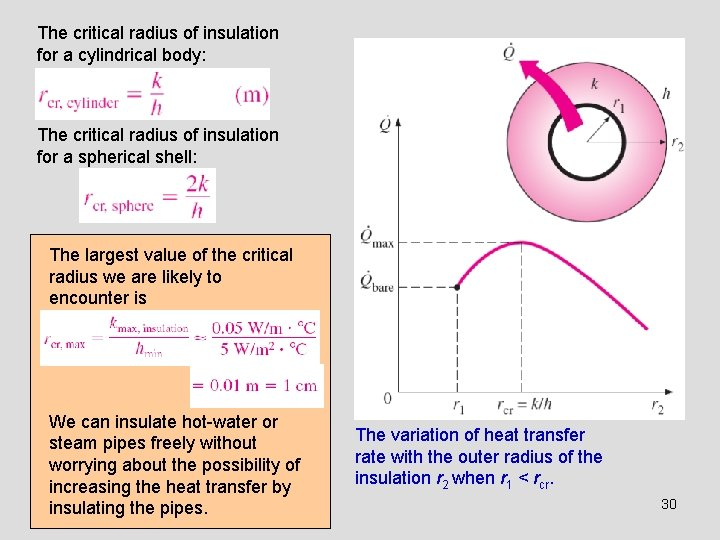The critical radius of insulation for a cylindrical body: The critical radius of insulation for a spherical shell: The largest value of the critical radius we are likely to encounter is We can insulate hot-water or steam pipes freely without worrying about the possibility of increasing the heat transfer by insulating the pipes. The variation of heat transfer rate with the outer radius of the insulation r 2 when r 1 < rcr. 30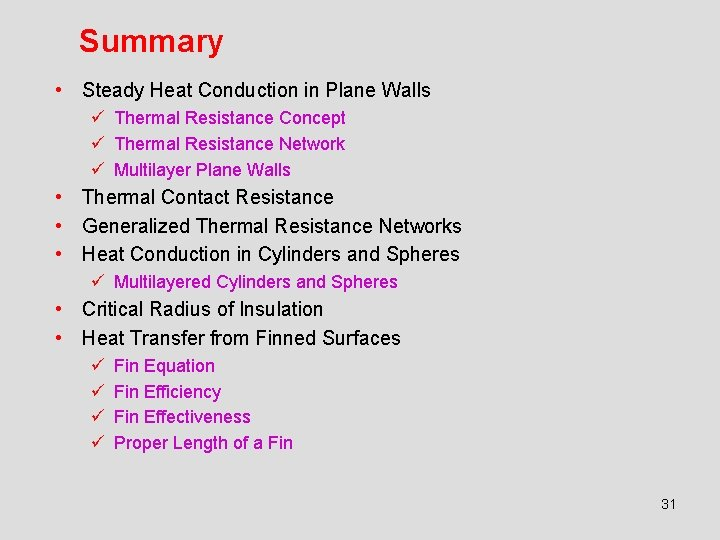Summary • Steady Heat Conduction in Plane Walls ü Thermal Resistance Concept ü Thermal Resistance Network ü Multilayer Plane Walls • Thermal Contact Resistance • Generalized Thermal Resistance Networks • Heat Conduction in Cylinders and Spheres ü Multilayered Cylinders and Spheres • Critical Radius of Insulation • Heat Transfer from Finned Surfaces ü ü Fin Equation Fin Efficiency Fin Effectiveness Proper Length of a Fin 31